# The Maxtrix Multiplicaiton Example

Matrix multiplication is a fundamental building block for scientific computing. Moreover, the algorithmic patterns of matrix multiplication are representative. Many other algorithms share similar optimization techniques as matrix multiplication. Therefore, matrix multiplication is one of the most important examples in learning parallel programming.

You can download the source code "5kk73matrixMul_cuda5.5.zip". Assume you copy code samples to your local directory under the folder `~/cuda/samples`. Then you can unzip the downloaded package to the folder `~/cuda-5.5/samples/0_Simple/5kk73matrixMul_cuda5.5`. You can compile this example using the Makefile in that folder, i.e., go to the folder and type the command "make". The commands are also shown below. Please first make sure you have set up the environment of the tools properly and can compile the official code examples.

Source code: 5kk73matrixMul_cuda5.5.zip

``````
cd ~/cuda-5.5/samples/0_Simple
unzip ./5kk73matrixMul_cuda5.5.zip
cd 5kk73matrixMul_cuda5.5
make
./5kk73matrixMul
```
```

## The Matrix Multiplicaiton Problem

Given a matrix A of size M x K and a matrix B of size K x N, multiply A with B and store the result into a matrix C of size M x N.

The matrixMul example on this page will show several techniques to optimize matrix multiplication on GPU. Most of them are generic, which can be applied to other applications. These techniques are as follows.

1. Tiling
2. Memory coalescing
3. Avoiding memory bank conflicts
4. Computation Optimizatio
5. Loop unrolling
6. Prefetching

The performance of these optimization techniques are show in the figures below (clink the figure to enlarge). Note: These optimizations, which are tuned for NVIDIA 8800 GT GPU at matrix size of 4096 x 4096, could be sub-optimal for other GPUs and other matrix sizes.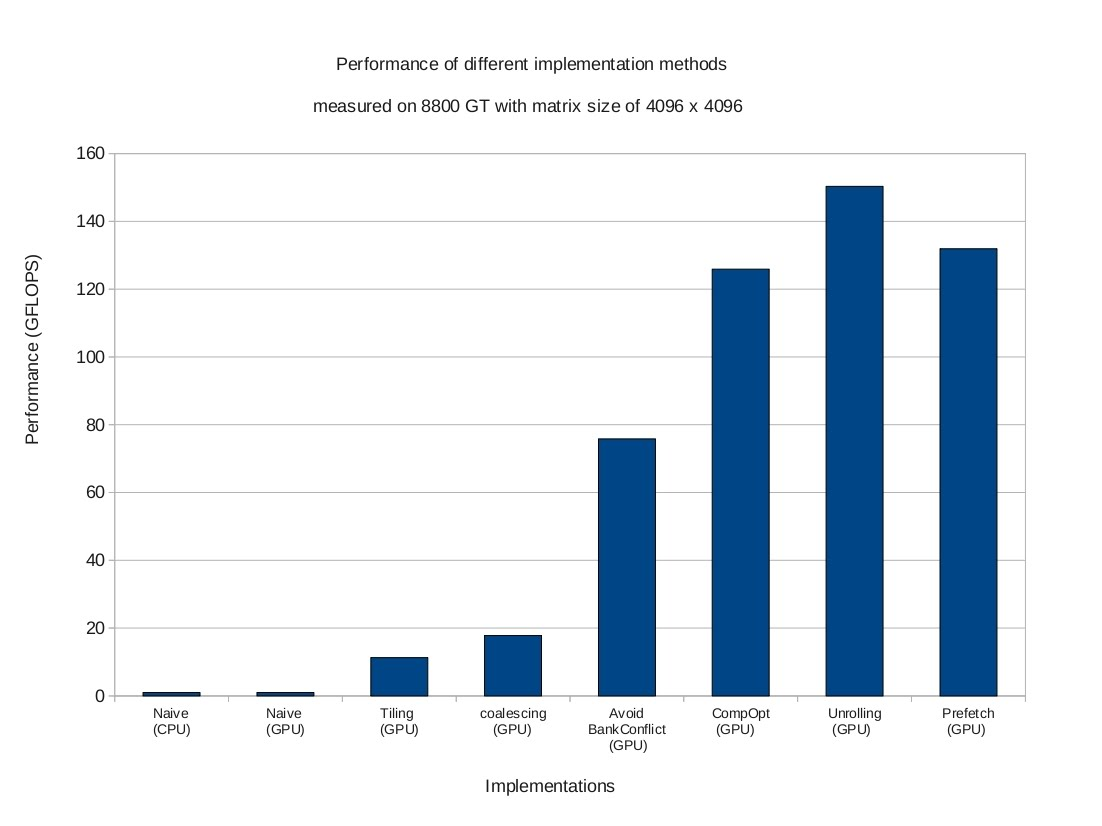We will start with a simple serial code running on CPU, and then go through these optimizations step by step.

## Naive Implementation On CPUs

``````
void main(){

define A, B, C

for i = 0 to M do
for j = 0 to N do
/* compute element C(i,j) */
for k = 0 to K do
C(i,j) <= C(i,j) + A(i,k) * B(k,j)
end
end
end
}
``````

To simplify the explanation, square matrices (M==N==K) are used in the illustrations. The figure below shows the memory footprint to compute an element C(3,11) (in red). This can be viewed as the inner product of one row of A (in blue) and one column of B (in green).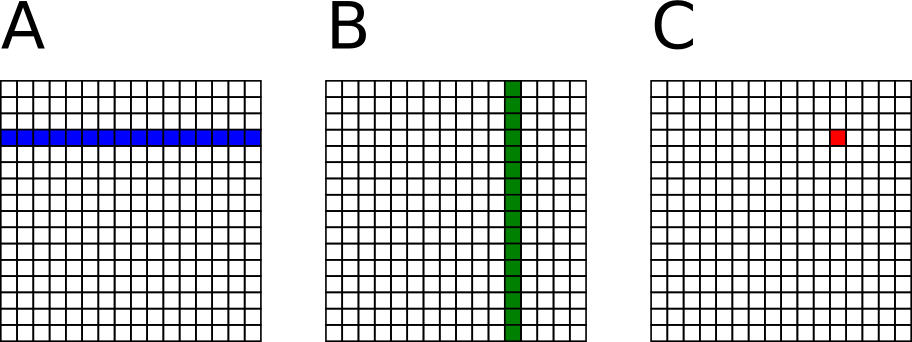## Naive Implementation On GPUs

``````
/* Codes running on CPU */

void main(){

define A_cpu, B_cpu, C_cpu in the CPU memory
define A_gpu, B_gpu, C_gpu in the GPU memory

memcopy A_cpu to A_gpu
memcopy B_cpu to B_gpu

dim3 dimBlock(16, 16)
dim3 dimGrid(N/dimBlock.x, M/dimBlock.y)

matrixMul<<< dimGrid, dimBlock >>>(A_gpu,B_gpu,C_gpu,K)

memcopy C_gpu to C_cpu

}
``````
``````
/* Codes running on GPU */

__global__ void matrixMul(A_gpu,B_gpu,C_gpu,K){

temp <= 0

i <= blockIdx.y * blockDim.y + threadIdx.y    // Row i of matrix C
j <= blockIdx.x * blockDim.x + threadIdx.x    // Column j of matrix C

for k = 0 to K-1 do
accu <= accu + A_gpu(i,k) * B_gpu(k,j)
end

C_gpu(i,j) <= accu

}
``````

A naive implementation on GPUs assigns one thread to compute one element of matrix C. Each thread loads one row of matrix A and one column of matrix B from global memory, do the inner product, and store the result back to matrix C in the global memory. The figure shows the memory footprint of one thread on global memory where matrix A, B, and C are stored.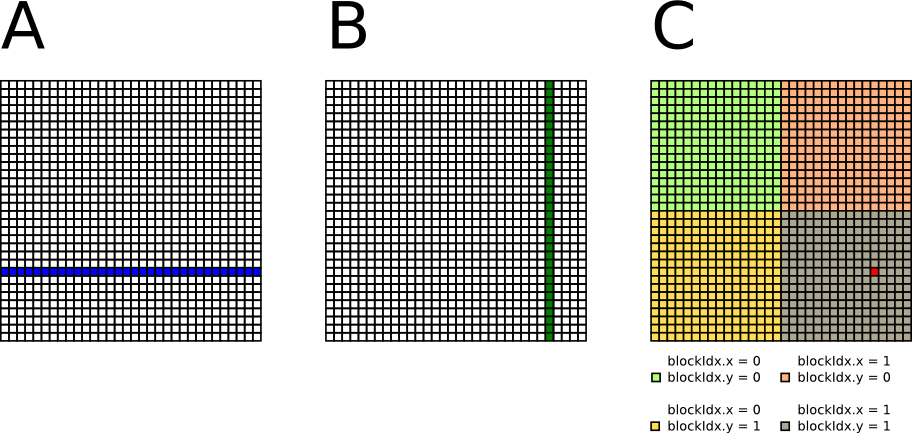In the naive implementation, the amount of computation is 2 x M x N x K flop, while the amount of global memory access is 2 x M x N x K word. The "computation-to-memory ratio" is approximately 1/4 (flop/byte). Therefore, the naive implementation is bandwidth bounded.

## Increasing "Computatin-to-Memory Ratio" by Tiling

To increase the "computation-to-memory ratio", the tiled matrix multiplication can be applied. One thread block computes one tile of matrix C. One thread in the thread block computes one element of the tile. The figure shows a 32 x 32 matrix divided into four 16 x 16 tiles. To compute this, four thread blocks each with 16 x 16 threads can be created.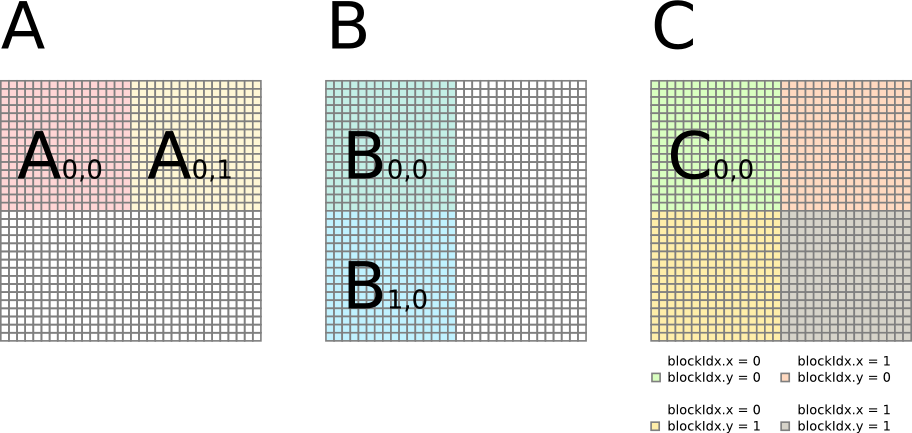The GPU kernel computes C in multiple iterations. In each iteration, one thread block loads one tile of A and one tile of B from global memory to shared memory, performs computation, and stores temporal result of C in register. After all the iteration is done, the thread block stores one tile of C into global memory. For example, a thread block can computer C(0,0) in two iterations: C(0,0) = A(0,0) B(0,0) + A(0,1) B(1,0).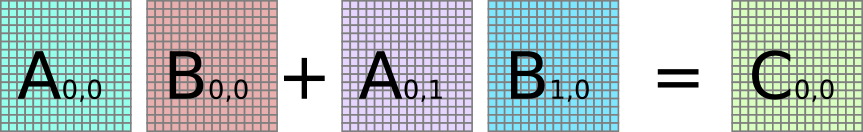In the first iteration, the thread block loads tile A0,0 and tile B0,0 from global memory into shared memory. Each thread performs inner product to produce one element of C. This element of C is stored in the register, which will be accumulated in the next iteration.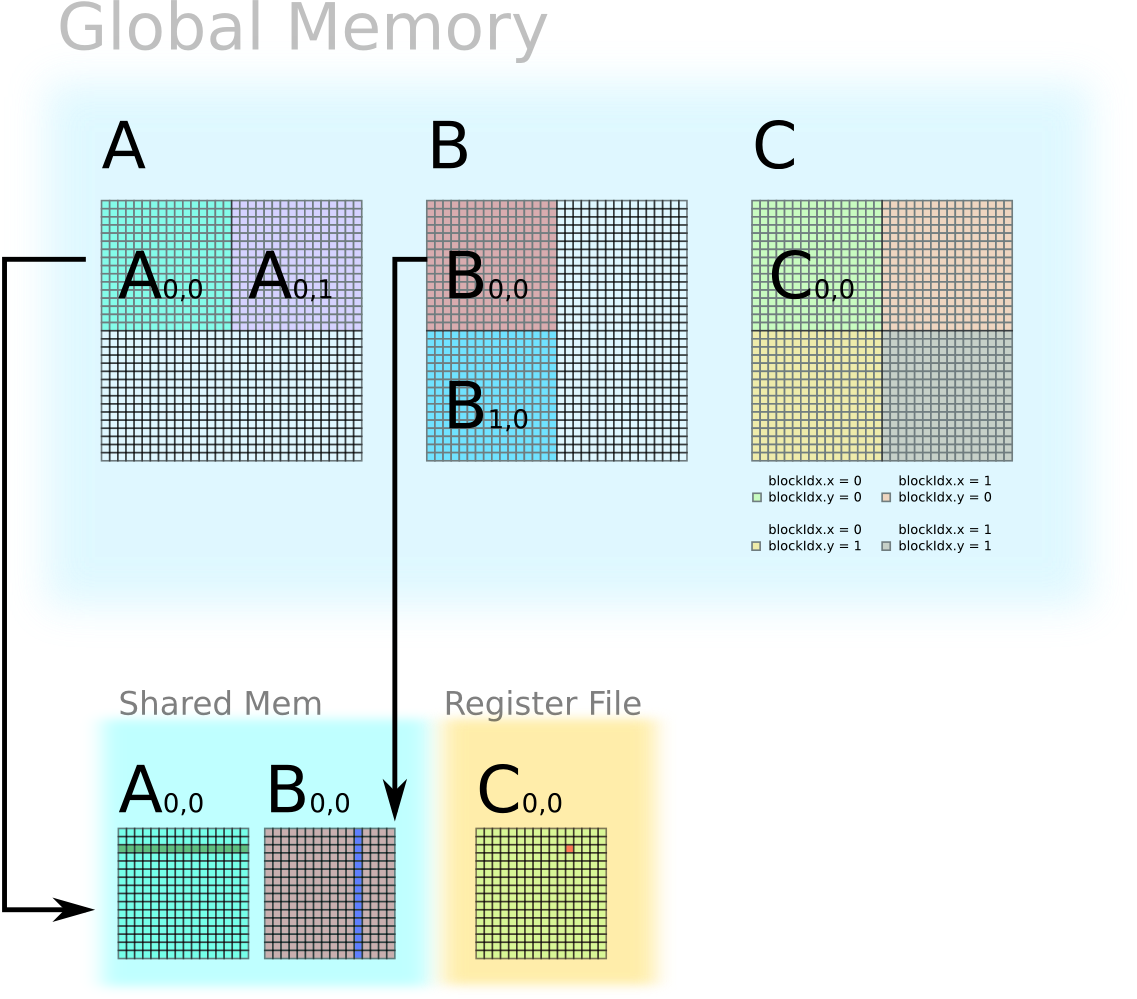In the second iteration, the thread block loads tile A(0,1) and tile B(1,0) from global memory into shared memory. Each thread performs the inner product to produce one element of C, which is accumulated with previous value. If this is the final iteration, then the element of C in the register file will be stored back into global memory.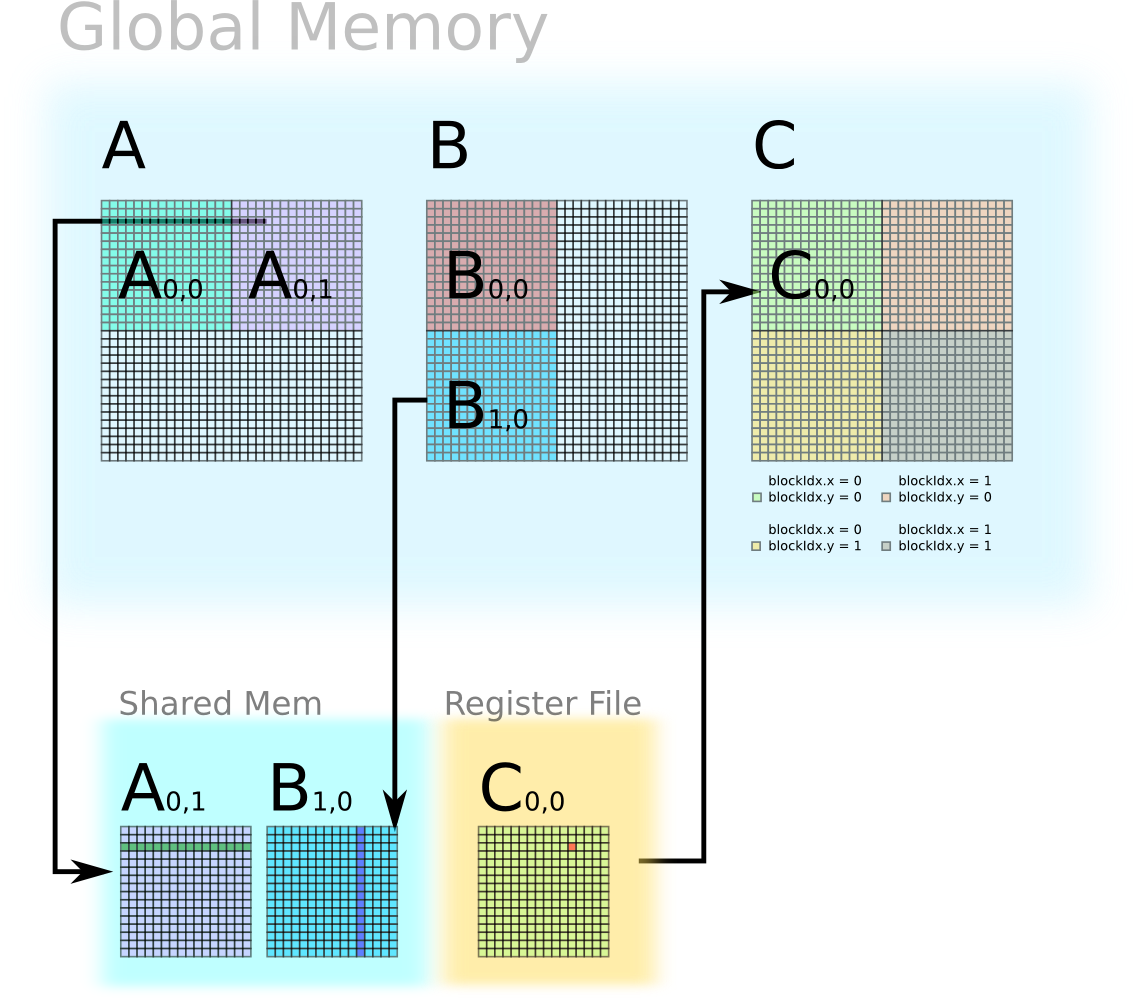The CPU code remains the same. Here only shows the GPU kernel.

``````
/* Codes running on GPU */

__global__ void matrixMul(A_gpu,B_gpu,C_gpu,K){

__shared__ float A_tile(blockDim.y, blockDim.x)
__shared__ float B_tile(blockDim.x, blockDim.y)

accu <= 0

/* Accumulate C tile by tile. */

for tileIdx = 0 to (K/blockDim.x - 1) do

/* Load one tile of A and one tile of B into shared mem */

// Row i of matrix A
i <= blockIdx.y * blockDim.y + threadIdx.y
// Column j of matrix A
j <= tileIdx * blockDim.x + threadIdx.x
// Load A(i,j) to shared mem
// Load B(j,i) to shared mem
// Synchronize before computation
__sync()

/* Accumulate one tile of C from tiles of A and B in shared mem */

for k = 0 to threadDim.x do
// Accumulate for matrix C
end
// Synchronize
__sync()

end

// Row i of matrix C
i <= blockIdx.y * blockDim.y + threadIdx.y
// Column j of matrix C
j <= blockIdx.x * blockDim.x + threadIdx.x
// Store accumulated value to C(i,j)
C_gpu(i,j) <= accu

}
``````

In the tiled implementation, the amount of computation is still 2 x M x N x K flop. However, using tile size of B, the amount of global memory access is 2 x M x N x K / B word. The "computation-to-memory ratio" is approximately B/4 (flop/byte). We now can tune the "computation-to-memory" ratio by changing the tile size B.

## Global Memory Coalescing

Two dimensional arrays in C/C++ are row-major. In the tiled implementation above, neighbouring threads have coalesced access to matrix A, but do not have coalesced access to matrix B. In column-major languages, such as Fortran, the problem is the other way around. An obvious solution is to transpose matrix B by CPU before offloading it to GPU memory.

``````
/* Codes running on GPU */

__global__ void matrixMul(A_gpu,B_gpu,C_gpu,K){

__shared__ float A_tile(blockDim.y, blockDim.x)
__shared__ float B_tile(blockDim.x, blockDim.y)

accu <= 0

/* Accumulate C tile by tile. */

for tileIdx = 0 to (K/blockDim.x - 1) do

/* Load one tile of A and one tile of B into shared mem */

// Row i of matrix A
i <= blockIdx.y * blockDim.y + threadIdx.y
// Column j of matrix A
j <= tileIdx * blockDim.x + threadIdx.x
// Load A(i,j) to shared mem
// Load B(i,j) to shared mem
// Synchronize before computation
__sync()

/* Accumulate one tile of C from tiles of A and B in shared mem */

for k = 0 to threadDim.x do
// Accumulate for matrix C    // Shared Mem Bank conflict
end
// Synchronize
__sync()

end

// Row i of matrix C
i <= blockIdx.y * blockDim.y + threadIdx.y
// Column j of matrix C
j <= blockIdx.x * blockDim.x + threadIdx.x
// Store accumulated value to C(i,j)
C_gpu(i,j) <= accu

}
``````

## Avoiding Shared Memory Bank Conflict

``````
/* Codes running on GPU */

__global__ void matrixMul(A_gpu,B_gpu,C_gpu,K){

__shared__ float A_tile(blockDim.y, blockDim.x)
__shared__ float B_tile(blockDim.x, blockDim.y)

accu <= 0

/* Accumulate C tile by tile. */

for tileIdx = 0 to (K/blockDim.x - 1) do

/* Load one tile of A and one tile of B into shared mem */

// Row i of matrix A
i <= blockIdx.y * blockDim.y + threadIdx.y
// Column j of matrix A
j <= tileIdx * blockDim.x + threadIdx.x
// Load A(i,j) to shared mem
// Load B(i,j) to shared mem
// Synchronize before computation
__sync()

/* Accumulate one tile of C from tiles of A and B in shared mem */

for k = 0 to threadDim.x do
// Accumulate for matrix C  // No Shared Mem Bank conflict
end
// Synchronize
__sync()

end

// Row i of matrix C
i <= blockIdx.y * blockDim.y + threadIdx.y
// Column j of matrix C
j <= blockIdx.x * blockDim.x + threadIdx.x
// Store accumulated value to C(i,j)
C_gpu(i,j) <= accu

}
``````

## Computation Optimization

After the aforementioned optimizations, the kernel is computation bound. Therefore, we need to increase the portion of useful floating point operation in total instructions. Because the inner product consumes most of the time, it is important to make sure this part is efficient. If we check the binary code for the inner product, we will discover one line of code in CUDA takes two instructions in the binary.

``````
/* CUDA code for inner product */

/* Disassembled from cubin binary */

mov.b32 \$r0, s[\$ofs4+0x0000]
``````

The current architecture of Stream Multiprocessor (SM) only allows one source operand from the shared memory. However, computing the inner product requires two source operands from from the shared memory. One solution is to store matrix A or matrix B into register file, but then the matrix in the register file can not be shared by different threads, which decreases the "computation-to-memory ratio".

A better solution is to perform outer product instead of inner product. In this case, matrix A is stored in shared memory, but matrix B and C are stored in registers. The outer product does not require sharing of matrix B and matrix C, therefore, each thread only stores one element of B and one column of the tile of C in the register. The "computation-to-memory ratio" of the outer product is the same as the inner product.

``````
/* CUDA code for outer product */
/* accu[i] and b are stored in register file */

accu[i] <= accu[i] + A_tile(i) * b

/* Disassembled From cubin binary */

``````

Here is an example of multiplying tile A(0,0) and tile B(0,0) to compute C(0,0) using outer product. In this example, A(0,0 is 16 x 16, B(0,0) is 16 x 64, C(0,0) is 16 x 64. A thread block of 64 threads is performing computing C(0,0).

### Step 1: load A(0,0) to shared memory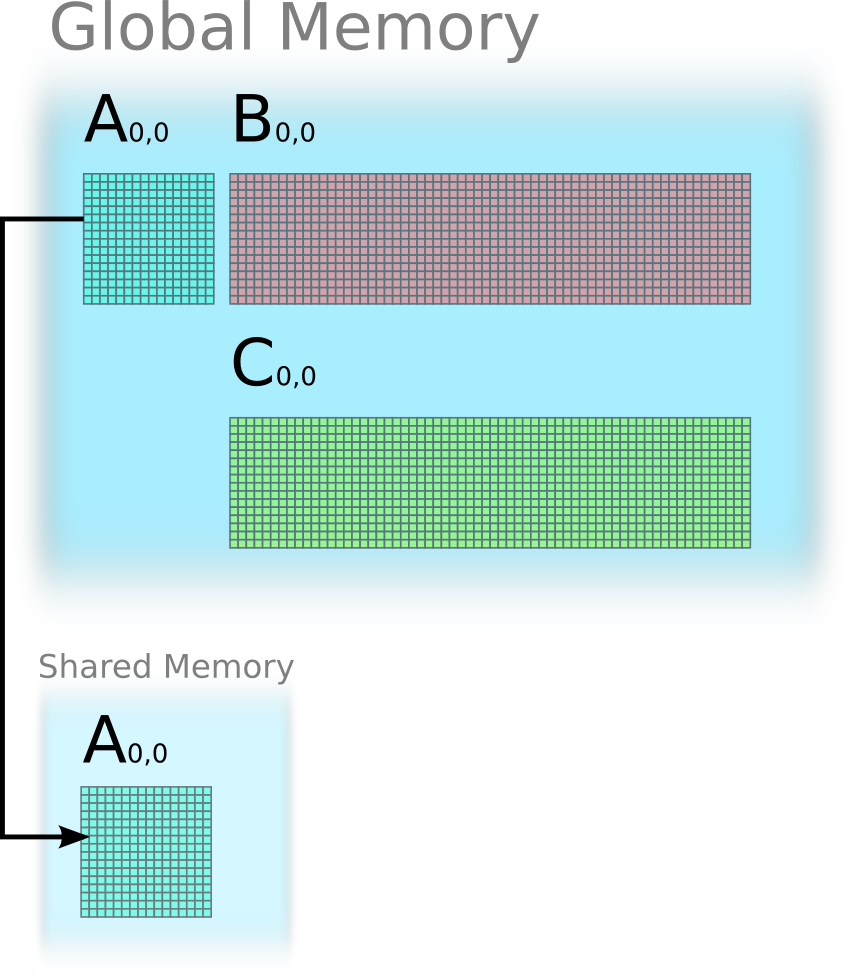### Step 2: use 16 iterations to update C(0,0)

Each thread stores one element of B(0,0) in its register. Each thread also stores one column of C(0,0) in its register.

Iteration 1: outer product between the first column of A(0,0) and the first row of B(0,0), and update C(0,0).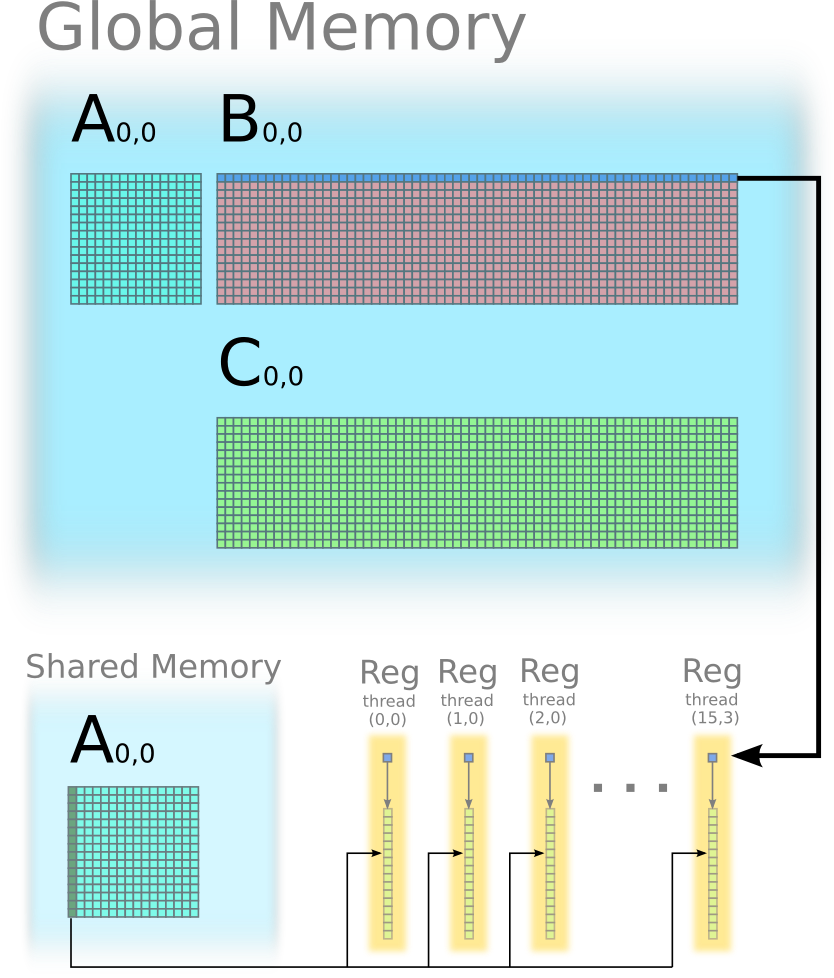Iteration 2: outer product between the second column of A(0,0) and the second row of B(0,0), and update C(0,0).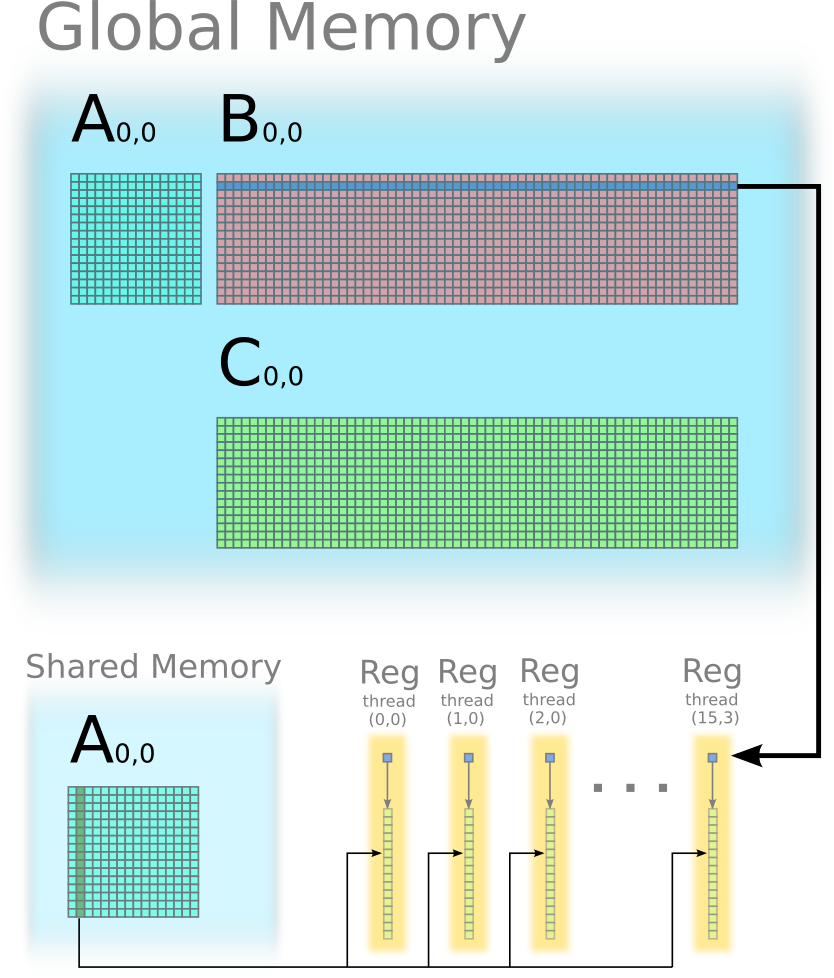Continue the iteration 3, 4, ..., 15 in similar way.

Iteration 16: outer product between the 16th column of A0,0 and the 16th row of B0,0, and update C0,0.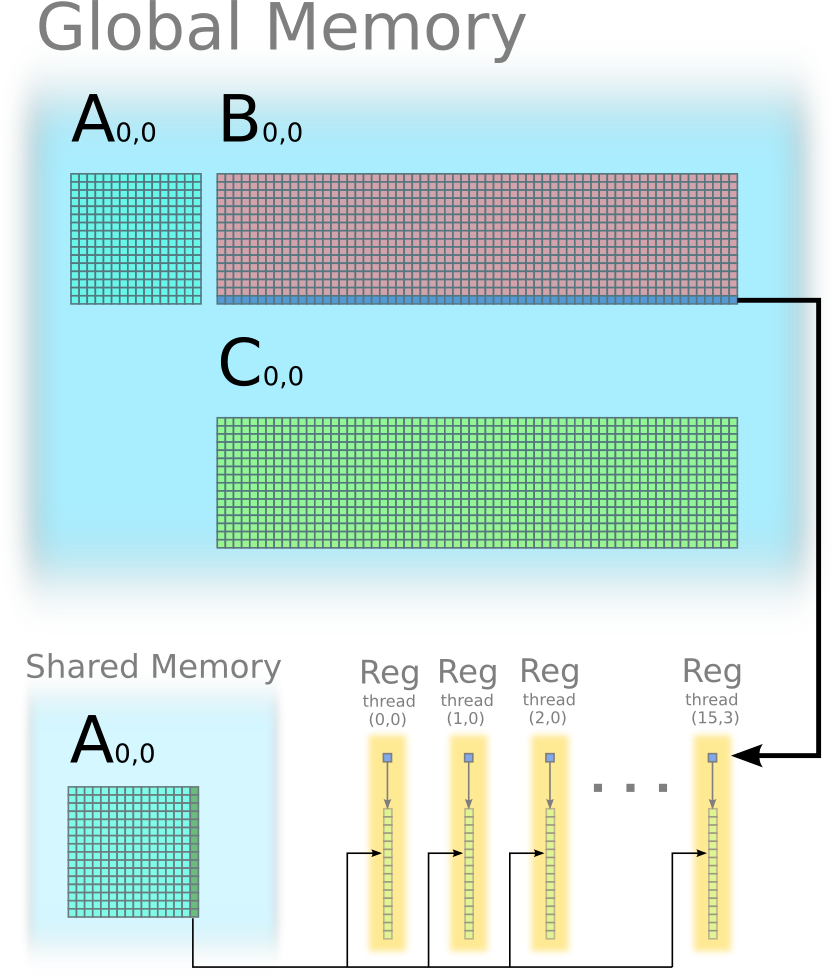### Step 3: each thread stores one column of C(0,0) from its register to global memory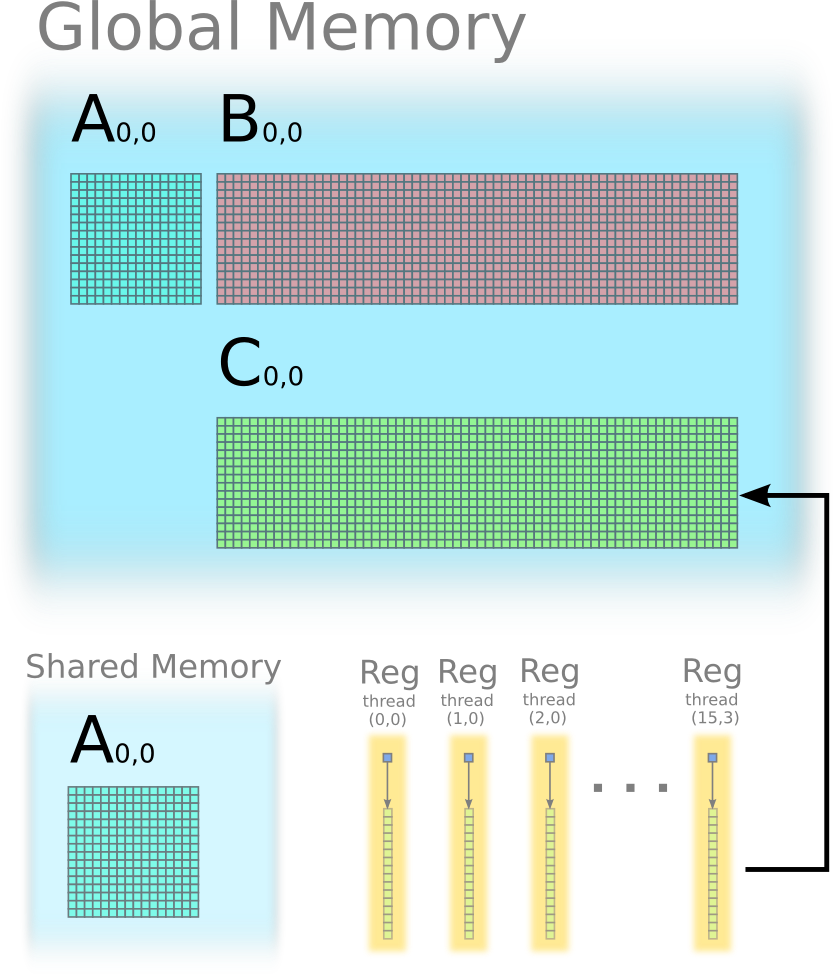## Loop Unrolling

Use the pragma to tell the compiler to unroll the loops. The nvcc will unroll the inner loops by default. But it will not unroll the outer loop unless told by the pragma.

``````
#pragma unroll
``````

Loop unrolling sometimes has side effects on register usage, which may limit the number of concurrent threads. However, the loop unrolling does not increase register usage in the matrixMul example.

## Prefetching

``````
/* Codes running on GPU */

__global__ void matrixMul(A_gpu,B_gpu,C_gpu,K){

__shared__ float A_tile0(blockDim.y, blockDim.x)
__shared__ float A_tile1(blockDim.x, blockDim.y)

float *pointer0 = A_tile0
float *pointer1 = A_tile1

fetch one tile of matrix A_gpu to pointer0

__sync()

/* Accumulate C tile by tile. */

for tileIdx = 0 to (K/blockDim.x - 1) do

prefetch one tile of matrix A_gpu to pointer1

accumulate C using pointer0

__sync()

swap pointer0 and pointer1

end

store tile C to global memory

}
``````